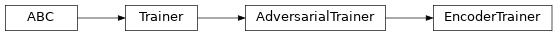# EncoderTrainer¶

Inheritance Diagramclass ashpy.trainers.EncoderTrainer(generator, discriminator, encoder, generator_optimizer, discriminator_optimizer, encoder_optimizer, generator_loss, discriminator_loss, encoder_loss, epochs, metrics=None, callbacks=None, logdir=PosixPath('/home/docs/checkouts/readthedocs.org/user_builds/ashpy/checkouts/latest/docs/source/log'), log_eval_mode=<LogEvalMode.TEST: 1>, global_step=None)[source]

Primitive Trainer for GANs using an Encoder sub-network.

The implementation is thought to be used with the BCE losses. To use another loss function consider subclassing the model and overriding the train_step method.

Examples

from pathlib import Path

import shutil
import operator

def real_gen():
label = 0
for _ in tf.range(100):
yield ((10.0,), (label,))

latent_dim = 100

generator = tf.keras.Sequential([tf.keras.layers.Dense(1)])

left_input = tf.keras.layers.Input(shape=(1,))
left = tf.keras.layers.Dense(10, activation=tf.nn.elu)(left_input)

right_input = tf.keras.layers.Input(shape=(latent_dim,))
right = tf.keras.layers.Dense(10, activation=tf.nn.elu)(right_input)

net = tf.keras.layers.Concatenate()([left, right])
out = tf.keras.layers.Dense(1)(net)

discriminator = tf.keras.Model(inputs=[left_input, right_input], outputs=[out])

encoder = tf.keras.Sequential([tf.keras.layers.Dense(latent_dim)])

# Losses
generator_bce = losses.gan.GeneratorBCE()
encoder_bce = losses.gan.EncoderBCE()
minmax = losses.gan.DiscriminatorMinMax()

epochs = 2

# Fake pre-trained classifier
num_classes = 1
classifier = tf.keras.Sequential(
[tf.keras.layers.Dense(10), tf.keras.layers.Dense(num_classes)]
)

if logdir.exists():
shutil.rmtree(logdir)

metrics = [metrics.gan.EncodingAccuracy(classifier)]

trainer = trainers.gan.EncoderTrainer(
generator=generator,
discriminator=discriminator,
encoder=encoder,
generator_loss=generator_bce,
discriminator_loss=minmax,
encoder_loss=encoder_bce,
epochs=epochs,
metrics=metrics,
logdir=logdir,
)

batch_size = 10
discriminator_input = tf.data.Dataset.from_generator(
real_gen, (tf.float32, tf.int64), ((1), (1))
).batch(batch_size)

dataset = discriminator_input.map(
lambda x, y: ((x, y), tf.random.normal(shape=(batch_size, latent_dim)))
)

trainer(dataset)

shutil.rmtree(logdir)

Initializing checkpoint.
Starting epoch 1.
Epoch 1 completed.
Starting epoch 2.
Epoch 2 completed.
Training finished after 2 epochs.


Methods

 __init__(generator, discriminator, encoder, …) Instantiate a EncoderTrainer. call(dataset[, log_freq, …]) Perform the adversarial training. train_step(real_xy, g_inputs) Adversarial training step.

Attributes

 ckpt_id_callbacks ckpt_id_discriminator ckpt_id_encoder ckpt_id_generator ckpt_id_global_step ckpt_id_optimizer_discriminator ckpt_id_optimizer_encoder ckpt_id_optimizer_generator ckpt_id_steps_per_epoch context Return the training context.
__init__(generator, discriminator, encoder, generator_optimizer, discriminator_optimizer, encoder_optimizer, generator_loss, discriminator_loss, encoder_loss, epochs, metrics=None, callbacks=None, logdir=PosixPath('/home/docs/checkouts/readthedocs.org/user_builds/ashpy/checkouts/latest/docs/source/log'), log_eval_mode=<LogEvalMode.TEST: 1>, global_step=None)[source]

Instantiate a EncoderTrainer.

Parameters: generator (tf.keras.Model) – A tf.keras.Model describing the Generator part of a GAN. discriminator (tf.keras.Model) – A tf.keras.Model describing the Discriminator part of a GAN. encoder (tf.keras.Model) – A tf.keras.Model describing the Encoder part of a GAN. generator_optimizer (tf.optimizers.Optimizer) – A tf.optimizers to use for the Generator. discriminator_optimizer (tf.optimizers.Optimizer) – A tf.optimizers to use for the Discriminator. encoder_optimizer (tf.optimizers.Optimizer) – A tf.optimizers to use for the Encoder. generator_loss (ashpy.losses.executor.Executor) – A ash Executor to compute the loss of the Generator. discriminator_loss (ashpy.losses.executor.Executor) – A ash Executor to compute the loss of the Discriminator. encoder_loss (ashpy.losses.executor.Executor) – A ash Executor to compute the loss of the Discriminator. epochs (int) – number of training epochs. metrics (Optional[List[Metric]]) – (List): list of ashpy.metrics.Metric to measure on training and validation data. callbacks (List) – List of ashpy.callbacks.Callback to call on events logdir (Union[Path, str]) – checkpoint and log directory. log_eval_mode (ashpy.modes.LogEvalMode) – models’ mode to use when evaluating and logging. global_step (Optional[tf.Variable]) – tf.Variable that keeps track of the training steps.
_build_and_restore_models(dataset)[source]

Build and restore a Subclassed model by firstly calling it on some data.

_train_step[source]

Perform the training step using the distribution strategy.

call(dataset, log_freq=10, measure_performance_freq=10)[source]

Parameters: dataset (tf.data.Dataset) – The adversarial training dataset. log_freq (int) – Specifies how many steps to run before logging the losses, e.g. log_frequency=10 logs every 10 steps of training. Pass log_frequency<=0 in case you don’t want to log. measure_performance_freq (int) – Specifies how many steps to run before measuring the performance, e.g. measure_performance_freq=10 measures performance every 10 steps of training. Pass measure_performance_freq<=0 in case you don’t want to measure performance.
train_step(real_xy, g_inputs)[source]